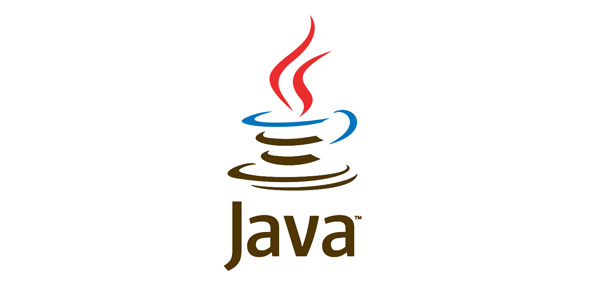# Java CIS 109 Test 1

14 Questions | Attempts: 299SettingsYou will be tested on java constructs such as loops, if conditions, methods etc

• 1.
Which is the correct boolean expression which gives the following meaning:The value of x is greater than 1000 and x is smaller or equal to 2000
• A.

X >= 1000 && x

• B.

X > 1000 && x < 2000

• C.

X < 1000 && x >= 2000

• D.

X > 1000 && x

• E.

None of the above

• 2.
Will this compile?class A{    System.out.println(20 * 10);}
• A.

Yes

• B.

No

• 3.
Will this compile?class B{   public static void main(String[] args)   {      Scanner sc = new Scanner(System.in);   }}
• A.

Yes

• B.

No

• 4.
What will this output?System.out.println(5/2);
• A.

2

• B.

2.5

• C.

Some kind of error

• D.

3

• 5.
What will this output?int i = 10;int j = 20;System.out.println("The result of "+j+" division by 2 is "+i+".");
• A.

The result of j division by 2 is i.

• B.

The result of 20 division by 2 is 10.

• C.

The result of10 division by 2 is 20.

• D.

None of the above

• 6.
What will this output?System.out.println(4 + 5 * 2);
• A.

14

• B.

18

• C.

None of the above

• 7.
Rewrite this as a for loopint i = 0;while (i < 10){   System.out.println(i);   i++;}
• 8.
Will the following compile?int i = 0;for (    ; i < 5 ;   ){   System.out.println(i);   i++;}
• A.

Yes

• B.

No

• 9.
What is the return types of the following body of a method{   int i = 10;   return 3 * i;}
• A.

Int

• B.

Long

• C.

Double

• D.

30

• E.

None of the above

• 10.
What is the return types of the following body of a method {    double d = 10;    return 3 * d; }
• A.

Int

• B.

Long

• C.

Double

• D.

30.0

• E.

None of the above

• 11.
What is the return types of the following body of a method {   return "test "+123; }
• A.

Int

• B.

Char

• C.

String

• D.

Test123

• E.

Will not compile

• 12.
What will the following output on the screen?for (int i = 0; i < 5; i++){   System.out.print("*");}
• A.

* * * * *

• B.

*****

• C.

******

• D.

* * * * * *

• E.

None of the above

• 13.
Write a program that will ask the user to start entering numbers. When he enters the number 0, you will show to him the sum of all the numbers he has entered.
• 14.
What will be the output of the following programclass A{   public static void main(String[] args)   {      System.out.println(getWelcomeMessage());   }   static String getWelcomeMessage()   {      return "Welcome!!!";   }}
• A.

GetWelcomeMessage()

• B.

GetWelcomeMessage

• C.

Welcome!!!

• D.

It would give a compilation error

## Related TopicsBack to top
×

Wait!
Here's an interesting quiz for you.